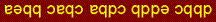CH223 - 11:00                            EXAM-1A                               Practice

 Information: gas constant:  R = 0.08206 L·atm/mole·K = 8.314 J/mole·K Avogadro's number:  NA = 6.022 ´ 1023/mol van't Hoff equation:  ln(K2/K1)= -(DH/R)(1/T2 -1/T1) normal freezing point of water:   0.00°C = 273.15K water ionization constant (25°C):  Kw = 1.00 ´ 10-14 pressure:  1 atm = 760 mmHg = 760 torr exp(x) = ex,  ln(ex) = x,  log(10x) = x quadratic equation:   ax2 + bx + c = 0,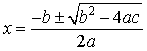Multiple choice:  select one answer for each question:

1.   Find the concentration of B in an equilibrium gas mixture that has [C] = 4.61 ´ 10-2 M:

B(g)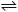2C(g) ,     Kc = 5.38 ´ 10-3

 a) 8.5 ´ 10-2 M b) 9.2 ´ 10-1 M c) 3.1 ´ 10-3 M d) 4.0 ´ 10-1 M e) 1.9 ´ 10-2 M

2.  The relationship between Kc and Kp for the reaction NO(g) + ½ O2(g)NO2(g) at 477ºC is

 a) Kc =  483 Kp b) Kc = 7.85 Kp c) Kc = 15.6 Kp d) Kc = 2.07 ´ 10-3  Kp e) Kc =  Kp

3.   If Kc = 0.145 for A2 + 2B2AB, then for ABB + ½ A2,  Kc would equal

 a) 0.381 b) 2.63 c) 0.145 d) -0.145 e) 6.90

4.   Predict the reaction direction when  [CS2] = [H2] = [CH4] = [H2S] = 0.125 M:

CS2(g) + 4 H2(g)CH4(g) + 2 H2S(g)  ,     Kc = 28

 a) reactants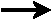products b) no net reaction, system is in equilibrium c) reactants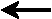products

5.  Which of these equilibria would be affected by pressure changes at constant temperature?

1.   FeO(s) + CO(g)Fe(s) + CO2(g)
2.   CaCO3(s)CaO(s) + CO2(g)
3.   2Mg(s) + CO2(g)2MgO(s) + C(s)

 a) 1 only b) 2 only c) 3 only d) 1 and 2 only e) 2 and 3 only

6. The reaction  5CO(g) + I2O5(g)I2(g) + 5CO2(g)  is exothermic.  The yield could be increased by

 a) increasing the pressure b) decreasing the pressure c) increasing the temperature d) decreasing the temperature e) decreasing the volume of the reaction vessel

7.   The symbol Kb(HCO3-) is the equilibrium constant for which reaction?

 a) HCO3- + OH-CO32- + H2O b) HCO3- + H2OCO32- + H3O+ c) HCO3- + H3O+H2CO3 + H2O d) HCO3- + H2OH2CO3 + OH- e) 2HCO3-H2CO3 + CO32-

8.  The pH of a solution that has [H3O+] = 5.12 × 10-3 M is:

 a) 6.4 b) 2.3 c) 5.1 d) 3.0

9. The Bronsted concept of acids and bases says the conjugate base of NH3 would be

 a) OH - b) NH4OH c) NH2- d) NH4+

10.  What is [OH-] in a solution that has [H3O+] = 3.16 × 10-5 M?

 a) 3.16 × 10-8 M b) 3.16 × 10-11 M c) 3.16 × 10-9 M d) 3.16 × 10-10 M

11.  The concentration of H3O+ in 0.44 M  hypobromous acid (HBrO, Ka = 2.3 ´ 10-9) is:

 a) 2.6 ´ 10-4 M b) 3.2 ´ 10-5 M c) 1.4 ´ 10-6 M d) 2.1 ´ 10-5 M

12.   What is the concentration of cyanide ion CN- in 0.003 M HCN (Ka = 6.2 × 10-10)?

 a) 1.4 ´ 10-6 M b) 6.2 ´ 10-10 M c) 8.4 ´ 10-7 M d) 2.5 ´ 10-5 M

13.   The pH of 0.033 M NH3 (Kb = 1.8 × 10-5) is

 a) 11.3 b) 9.25 c) 10.9 d) 12.8

14.  Which solution has the lowest pH?

 a) 0.10 M HNO2 b) 0.10 M HNO3 c) 0.10 M KNO2 d) 0.10 M KNO3

15.  The correct order of increasing base strength (weaker < stronger) is:

 a) BrO3- < BrO2- < BrO- b) BrO3- < BrO- < BrO2- c) BrO2- < BrO- < BrO3- d) BrO- < BrO2- < BrO3-

16.  Fe3+ in the reaction Fe3+ + 6 H2OFe(H2O)63+ is best described as a

 a) Bronsted acid b) Bronsted base c) Lewis acid d) Lewis base e) Arrhenius acid

17.  How does the acid equilibrium shift after some sodium acetate salt (CH3COONa ) is added to a solution of acetic acid (CH3COOH, Ka = 1.8 ´ 10-5)?

 a) CH3COOH + H2OH3O+ + CH3COO-   (pH increases) b) CH3COOH + H2OH3O+ + CH3COO-   (pH increases) c) CH3COOH + H2OH3O+ + CH3COO-   (pH decreases) d) CH3COOH + H2OH3O+ + CH3COO-   (pH decreases)

18.   Use the acid constants for HCN (Ka = 6 ´ 10-10) and HClO (Ka = 3 ´ 10-8)  to calculate Kc for

ClO-(aq) + HCN(aq)CN-(aq) + HClO(aq)

 a) 2 ´ 10-3 b) 2 ´ 10-2 c) 2 ´ 10-4 d) 2 ´ 103 e) 2 ´ 1012

19.  A diprotic acid H2A has values of Ka1 = 1.0 ´ 10-6 and Ka2 = 1.0 ´ 10-10.  What is [A2-] in a 0.10 M solution of H2A?

 a) 0.10 M b) 0.20 M c) 3.2 ´ 10-4 d) 3.2 ´ 10-6 e) 1.0 ´ 10-10

20.  Calculate the degree of ionization in 0.72 M acid HA (Ka = 0.12).   HINT: use the quadratic equation.

 a) 33% b) 50% c) 75% d) 25% e) 67%

 KEY: